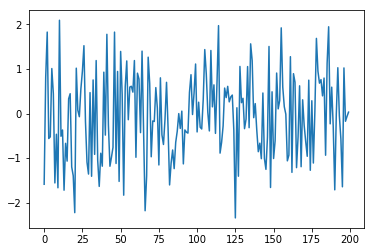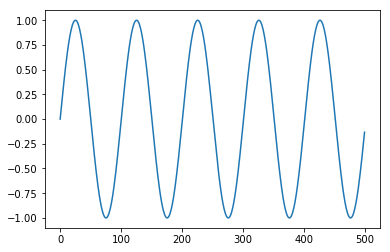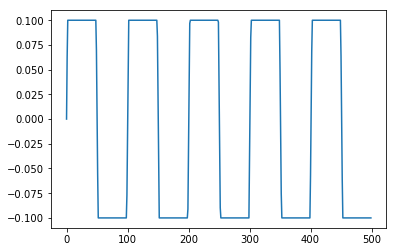Sound Demo 2

Michael Lamoureux
In :
%matplotlib inline
from matplotlib.pyplot import *
from numpy import *    ## for numerical functions
from IPython.display import Audio  ## to output audio


## What is sound?¶

Rapid changes, or vibrations in air pressure are heard in our ears as sound.

A computer creates sound by sending a list of numbers to a speaker, which then vibrates.

## Random numbers¶

A list of random numbers, will create a sound.

What do you think is will sound like?

In :
Fs = 44100
random_sd = random.randn(Fs)

In :
## Random sound

Audio(data=random_sd, rate=Fs)

Out:

We can plot a few of those numbers, using a plotting command.

In :
plot(random_sd[0:200]);## Periodic vibrations¶

A vibration that has a periodic vibration will sound like a tone to us.

The sine function has a periodic cycle, and can be used to represent such a tone.

In :
Fs, Len, f1 = 44100, 3, 440  ## sample rate, length in second, frequency
t = linspace(0,Len,Fs*Len)  ## time variable

In :
sine_sd = sin(2*pi*440*t)

Audio(data=sine_sd, rate=Fs)

Out:

We can plot this sine sound, using a plotting command.

In :
plot(sine_sd[0:500]);By clipping the tops of the sine wave, we get a square wave.

In :
square_sd = minimum(.1, maximum(-.1,sine_sd))
plot(square_sd[0:500]);In :
## What does a square wave sound like?

Audio(data=square_sd, rate=Fs)

Out:

The Sine and Square waves have the same pitch (frequency) but different timbres.

## Beats¶

Playing two tones at similar frequencies results in beats.

This is useful for tuning instruments!

In :
sine2_sd = sin(2*pi*440*t) - sin(2*pi*442*t)

Audio(data=sine2_sd, rate=Fs)

Out: